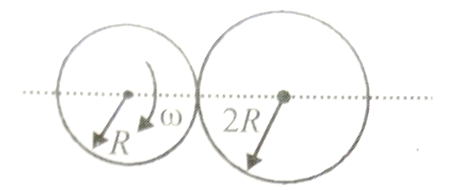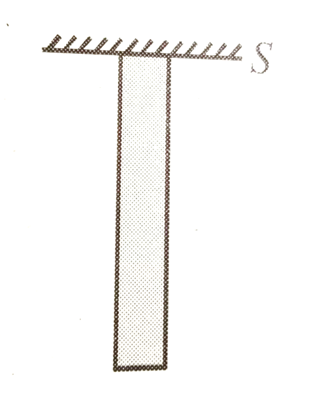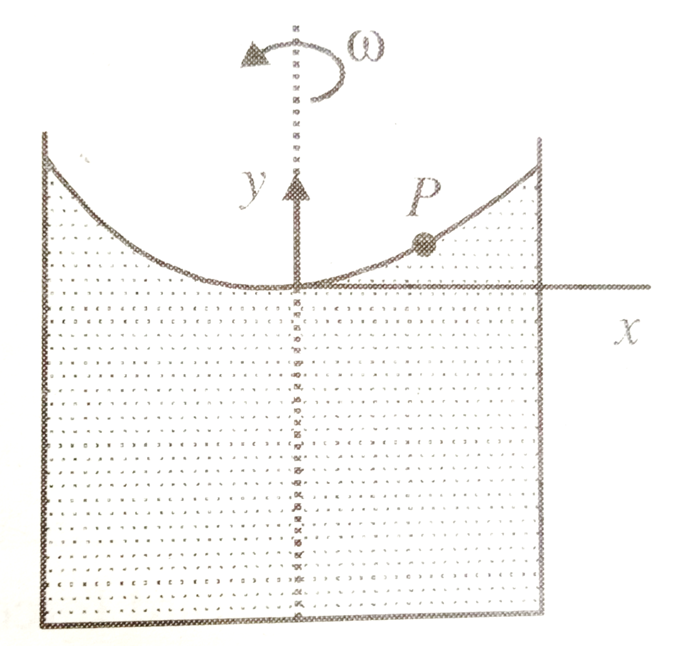A long spring is stretched by 2 cm, its potential energy is U. If the spring is streched by 10 cm, find the potential energy stored in it.   [This question is only for Dropper and XII batch]

1. 10 U

2. 15 U

3. 20 U

4. 25 U

Concept Questions :-

Integration
High Yielding Test Series + Question Bank - NEET 2020

Difficulty Level:

A spring of spring constant  is stretched initially by 5 cm from the unstretched position. Find the work required to stretch it further by another 5 cm is   [This question is only for Dropper and XII batch]

1. 15 J

2. 18.75 N .m

3. 20 J

4. 22.75 N .m

Concept Questions :-

Integration
High Yielding Test Series + Question Bank - NEET 2020

Difficulty Level:

An automobile of mass m accelerates, starting from rest, while the engine supplies constant power P, its position and velocity changes w.r.t time as-    [This question is only for Dropper and XII batch]

1.

2.

3.

4.

Concept Questions :-

Integration
High Yielding Test Series + Question Bank - NEET 2020

Difficulty Level:

A constant force F is applied on a body. The power (P) generated is related to the time elapsed (t) as

[This question is only for Dropper and XII batch]

1.

2.

3.

4.

Concept Questions :-

Integration
High Yielding Test Series + Question Bank - NEET 2020

Difficulty Level:

A rod of length L is placed along the x-axis between x= 0 and x= L. The linear density (mass/length)$\lambda$ of the rod varies with the distance x from the origin as $\lambda$= Rx. Here, R is a positive constant. Find the position of centre of mass of this rod.

[This question is only for Dropper and XII batch]

1.

2.

3.

4.

Concept Questions :-

Integration
High Yielding Test Series + Question Bank - NEET 2020

Difficulty Level:

A wheel rotates with an angular acceleration . Here t is the time and a, b are constants. If the wheel has an initial angular velocity ${\omega }_{0}$, find (a) the angular velocity and (b) the angle turned as function of time

[This question is only for Dropper and XII batch]

Concept Questions :-

Integration
High Yielding Test Series + Question Bank - NEET 2020

Difficulty Level:

Two discs of radii R and 2R are pressed against each other. Initially disc with radius R is rotating with angular velocity $\omega$ and other disc was stationary. Both disc are hinged at their respective centres and free to rotate about them.Moment of inertia of smaller is I and bigger disc is 2I about their respective axis of rotation. Find the angular velocity of the bigger disc after long time.

[This question is only for Dropper and XII batch]1. $\omega$

2. $\omega$/2

3. $\omega$/3

4. $\frac{2\omega }{3}$

Concept Questions :-

Integration
High Yielding Test Series + Question Bank - NEET 2020

Difficulty Level:

The gravitational field due to a mass distribution is given by , where k is a constant. Assuming the potential to be zero at infinity, find the potential at a point x = a. [This question is only for Dropper and XII batch]

1. $\frac{k}{{a}^{2}}$

2. $\frac{-k}{{a}^{2}}$

3. $\frac{k}{a}$

4. $\frac{-k}{a}$

Concept Questions :-

Integration
High Yielding Test Series + Question Bank - NEET 2020

Difficulty Level:

A bar of mass M and length L is hanging from point S as shown in figure. The Young's modulus of elasticity of the wire is Y and the area of cross-section of the wire is A. Find total elongation in bar.

[This question is only for Dropper and XII batch]1. $\frac{MgL}{AY}$

2. $\frac{MgL}{2AY}$

3. $\frac{2MgL}{AY}$

4. $\frac{3MgL}{2AY}$

Concept Questions :-

Integration
High Yielding Test Series + Question Bank - NEET 2020

Difficulty Level:

Consider a liquid of density $\rho$ in a container that spins with angular velocity $\omega$ as shown in figure. Find relation between y and x for any point P, if liquid rises due to rotation. [This question is only for Dropper and XII batch]1. $y=\frac{\omega x}{2g}$

2. $y=\frac{{\omega }^{2}{x}^{2}}{2g}$

3. $y=\frac{{\omega }^{2}x}{2g}$

4. $y=\frac{\omega {x}^{2}}{2g}$

Concept Questions :-

Integration